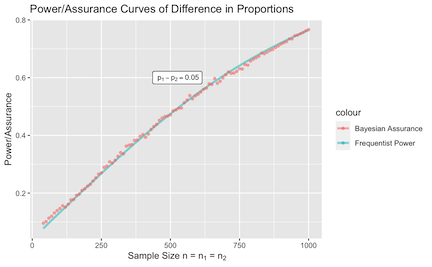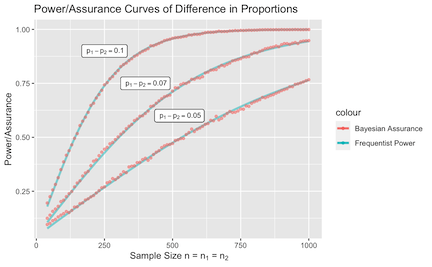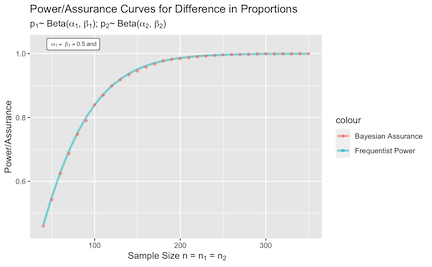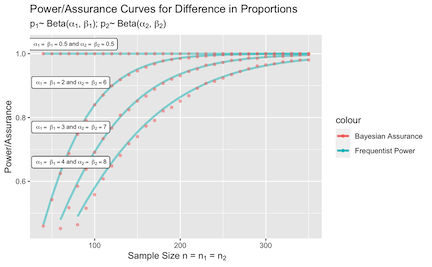# 1 Sample Size Determination Under Precision-Based Conditions

The bayes_adcock() function determines the assurance under precision-based conditions discussed in Adcock (1997). Recall in the frequentist setting, if $$X_i \sim N(\theta, \sigma^2)$$ for $$i = 1,..., n$$ observations and variance $$\sigma^2$$ is known, the precision can be calculated using $$d = z_{1-\alpha/2}\frac{\sigma}{\sqrt{n}}$$, where $$z_{1-\alpha/2}$$ is the critical value for the $$100(1-\alpha/2)\%$$ quartile of the standard normal distribution. Simple rearranging leads to the following expression for sample size: $\begin{equation} n = z_{1-\alpha/2}^2\frac{\sigma^2}{d^2}\;. \end{equation}$

Analysis Stage

Given a random sample with mean $$\bar{x}$$, suppose the goal is to estimate population mean $$\theta$$.

To formulate this problem in the Bayesian setting, suppose $$x_1, \cdots, x_n$$ is a random sample from $$N(\theta, \sigma^2)$$ and the sample mean is distributed as $$\bar{x}|\theta, \sigma^2 \sim N(\theta, \sigma^2/n)$$. The analysis objective is to observe that the absolute difference between $$\bar{x}$$ and $$\theta$$ falls within a margin of error no greater than $$d$$. Given data $$\bar{x}$$ and a pre-specified confidence level $$\alpha$$, the assurance can be formally expressed as $\begin{equation} \delta = P_{\bar{x}}\{\bar{x}: P(|\bar{x} - \theta| \leq d) \geq 1-\alpha\}\;. \end{equation}$ We assign $$\theta \sim N(\theta_0^{(a)}, \sigma^2/n_a)$$ as the analysis prior, where $$n_a$$ quantifies the amount of prior information we have for $$\theta$$. The posterior of $$\theta$$ is obtained by taking the product of the prior and likelihood, resulting in $\begin{equation} N\Bigg(\bar{x} {\left| \theta, \frac{\sigma^2}{n}\right.}\Bigg) \times N\Bigg(\theta {\left | \theta_0^{(a)}, \frac{\sigma^2}{n_a}\right.}\Bigg) = N\Bigg(\theta {\left | \lambda, \frac{\sigma^2}{n_a+n}\right.}\Bigg), \end{equation}$ where $$\lambda = \frac{n\bar{x}+n_a\theta_0^{(a)}}{n_a+n}$$. From here we can further evaluate the condition using parameters from the posterior of $$\theta$$ to obtain a more explicit version of the analysis stage objective. From $$P(|\bar{x} - \theta| \leq d) = P(\bar{x} - d \leq \theta \leq \bar{x} + d)$$, we can standardize all components of the inequality using the posterior distribution of $$\theta$$, eventually leading to $\begin{equation} \label{eq:adcock_region_simplified} \delta = \left\{ \bar{x}: \Phi\left[\frac{\sqrt{n_a + n}}{\sigma} (\bar{x} + d - \lambda)\right] - \Phi\left[\frac{\sqrt{n_a+n}}{\sigma}(\bar{x} - d - \lambda)\right] \geq 1-\alpha \right\}. \end{equation}$

Design Stage

In the design stage, we need to construct a protocol for sampling data that will be used to evaluate the analysis objective. This is achieved by first setting a separate design stage prior on $$\theta$$ such that $$\theta \sim N(\theta_0^{(d)}, \sigma^2/n_d)$$, where $$n_d$$ quantifies our degree of belief towards the population from which the sample will be drawn. Given that $$\bar{x}|\theta, \sigma^2 \sim N(\theta, \sigma^2/n)$$, the marginal distribution of $$\bar{x}$$ can be computed using straightforward substitution based on $$\bar{x} = \theta + \epsilon;$$ $$\epsilon \sim N(0, \sigma^2/n)$$ and $$\theta = \theta_0^{(d)} + \omega;$$ $$\omega \sim N(0, \sigma^2/n_d)$$.
Substitution $$\theta$$ into the expression for $$\bar{x}$$ gives us $$\bar{x}=\theta_0^{(d)} + (\omega + \epsilon);$$ $$(\omega + \epsilon) \sim N\Big(0, \frac{\sigma^2(n_d+n)}{n_dn}\Big) = N(0, \sigma^2/p)$$, where $$1/p = 1/n_d + 1/n$$. The marginal of $$\bar{x}$$ is therefore $$N(\bar{x}|\theta_0^{(d)}, \sigma^2/p)$$, where we will be iteratively drawing samples from to check if they satisfy the condition outlined in the analysis stage.

## 1.1 Example

First, load in the bayesassurance package.

library(bayesassurance)

Specify the following inputs:

1. n: sample size (either vector or scalar).
2. d: precision level in in analysis objective $$|\bar{x} - \theta| \leq d$$
3. mu_beta_a: analysis stage mean
4. mu_beta_d: design stage mean
5. n_a: sample size at analysis stage. Quantifies the amount of prior information we have for parameter $$\theta$$.
6. n_d: sample size at design stage. Quantifies the amount of prior information we have for where the data is being generated from.
7. sig_sq: known variance $$\sigma^2$$.
8. alpha: significance level
9. mc_iter: number of MC samples evaluated under the analysis objective.

As an example, we assign the following set of arbitrary inputs to pass into bayes_adcock() and save the output as out. Notice that we assign a vector of values to sample size n and choose arbitrary values for the remaining parameters.

library(ggplot2)
n <- seq(20, 145, 5)

set.seed(20)
out <- bayesassurance::bayes_adcock(n = n, d = 0.20, mu_beta_a = 0.64, mu_beta_d = 0.9,
n_a = 20, n_d = 10, sig_sq = 0.265, alpha = 0.05,
mc_iter = 10000)

Within out contains a list of three objects:

1. assurance_table: table of sample sizes and corresponding assurance values.
2. assur_plot: plot of assurance values. Returned if length of n is greater than 1.
3. mc_iter: number of MC samples generated.

The first six entries of the resulting power table is shown by calling head(out$assurance_table). head(out$assurance_table)
n Assurance
20 0.2378
25 0.3009
30 0.3664
35 0.4376
40 0.5267
45 0.5981

The plot of assurance points is produced using the ggplot2 package. It displays the inputted sample sizes on the x-axis and the resulting assurance values on the y-axis.

n1 n2 Assurance
600 600 0.5482
610 610 0.5564
620 620 0.5662
630 630 0.5616
640 640 0.5736
650 650 0.5840

## 2.4 Overlapping Behaviors Between Frequentist and Bayesian Settings

We will demonstrate cases where the Bayesian and frequentist settings align and perform similarly in behavior.

Case 1: Known Probabilities

If $$p_1$$ and $$p_2$$ are known, there is no need rely on the Beta distribution to randomly generate values. We still, however, need to assign shape parameters in the Bayesian setting as they are needed to compute the posterior credible intervals. We will use Haldane’s priors (flat priors) for the Beta distribution, in which $$\alpha$$ and $$\beta$$ are set to 0.5.

Recall the sample size formula for assessing differences in proportions in the frequentist setting, $n = \frac{(z_{1-\alpha/2} + z_{\beta})^2(p_1(1-p_1) + p_2(1-p_2))}{(p_1 - p_2)^2},$ where $$n = n_1 = n_2$$. Simple rearragements and noting that $$-(z_{1-\alpha/2} + z_{\beta}) = z_{1-\beta} - z_{1-\alpha/2}$$ lead us to obtain $\begin{multline*} %\frac{\sqrt{n}(p_1 - p_2)}{\sqrt{p_1(1-p_1) + p_2(1-p_2)}} = z_{1-\beta} - z_{1-\alpha/2} \frac{\sqrt{n}(p_1 - p_2)}{\sqrt{p_1(1-p_1) + p_2(1-p_2)}} + z_{1-\alpha/2} = z_{1-\beta} \implies \text{Power} = 1 - \beta = \\ \Phi\left(\frac{\sqrt{n}(p_1 - p_2)}{\sqrt{p_1(1-p_1) + p_2(1-p_2)}} + z_{1-\alpha/2}\right). \end{multline*}$

We create a simple function corresponding to this power formula:

propdiffCI_classic <- function(n, p1, p2, sig_level){
p <- p1 - p2
power <- pnorm(sqrt(n / ((p1*(1-p1)+p2*(1-p2)) / (p)^2)) - qnorm(1-sig_level/2))
return(power)
}

We define a vector of sample sizes $$n$$ that are assigned to both $$n_1$$ and $$n_2$$ in the Bayesian setting. Arbitrary values are assigned to $$p_1$$ and $$p_2$$ along with vague shape parameters, i.e.  $$\alpha_1 = \alpha_2 = \beta_1 = \beta_2 = 0.5$$. We plot the power curve and assurance points on the same plot.

#########################################################
# alpha1 = 0.5, beta1 = 0.5, alpha2 = 0.5, beta2 = 0.5 ##
#########################################################
n <- seq(40, 1000, 10)

power_vals <- sapply(n, function(i) propdiffCI_classic(n=i, 0.25, 0.2, 0.05))
df1 <- as.data.frame(cbind(n, power_vals))

assurance_out <- bayes_sim_betabin(n1 = n, n2 = n, p1 = 0.25,
p2 = 0.2, alpha_1 = 0.5, beta_1 = 0.5, alpha_2 = 0.5, beta_2 = 0.5,
sig_level = 0.05, alt = "two.sided")
df2 <- assurance_out$assurance_table colnames(df2) <- c("n1", "n2", "Assurance") p1 <- ggplot(df1, alpha = 0.5, aes(x = n, y = power_vals, color="Frequentist Power")) p1 <- p1 + geom_line(alpha = 0.5, aes(x = n, y = power_vals, color="Frequentist Power"), lwd = 1.2) p2 <- p1 + geom_point(data = df2, alpha = 0.5, aes(x = .data$n1, y = .data$Assurance, color = "Bayesian Assurance"), lwd = 1.2) + ylab("Power/Assurance") + xlab(~ paste("Sample Size n = ", "n", " = ", "n")) + ggtitle("Power/Assurance Curves of Difference in Proportions") p2 <- p2 + geom_label(aes(525, 0.6, #label="~alpha == ~beta == 0.5~and~alpha == ~beta == 0.5"), label = "~p-p == 0.05"), parse = TRUE, color = "black", size = 3)Notice that the power and simulated assurance points overlap. This relies on the fact that the normal distribution can be used to approximate binomial distributions for large sample sizes given that the Beta distribution is approximately normal when its parameters $$\alpha$$ and $$\beta$$ are set to be equal. We repeat these steps for different $$p_1$$ and $$p_2$$ values while maintaining the same shape parameters.Case 2: Unknown Probabilities The following code segments are plots demonstrate overlapping behaviors of the frequentist and Bayesian settings when $$p_1$$ and $$p_2$$ are not known. We try this for various shape parameters, recalling the fact that the normal distribution can be used to approximate binomial distributions for large sample sizes given that the Beta distribution is approximately normal when its parameters $$\alpha$$ and $$\beta$$ are set to be equal. We will modify the frequentist power function to include shape parameters as inputs. propdiffCI_classic <- function(n, p1, p2, alpha_1, beta_1, alpha_2, beta_2, sig_level){ set.seed(1) if(is.null(p1) == TRUE & is.null(p2) == TRUE){ p1 <- rbeta(n=1, alpha_1, beta_1) p2 <- rbeta(n=1, alpha_2, beta_2) }else if(is.null(p1) == TRUE & is.null(p2) == FALSE){ p1 <- rbeta(n=1, alpha_1, beta_1) }else if(is.null(p1) == FALSE & is.null(p2) == TRUE){ p2 <- rbeta(n=1, alpha_2, beta_2) } p <- p1 - p2 power <- pnorm(sqrt(n / ((p1*(1-p1)+p2*(1-p2)) / (p)^2)) - qnorm(1-sig_level/2)) return(power) } The following code chunk assigns NULL values to $$p_1$$ and $$p_2$$ in both functions and assigns equal sets of shape parameters for the Beta distributions. ################################################ # alpha1 = 2, beta1 = 2, alpha2 = 6, beta2 = 6 # ################################################ library(ggplot2) power_vals <- propdiffCI_classic(n=n, p1 = NULL, p2 = NULL, 2, 2, 6, 6, 0.05) df1 <- as.data.frame(cbind(n, power_vals)) assurance_vals <- bayes_sim_betabin(n1 = n, n2 = n, p1 = NULL, p2 = NULL, alpha_1 = 2, beta_1 = 2, alpha_2 = 6, beta_2 = 6, sig_level = 0.05, alt = "two.sided") df2 <- assurance_vals$assurance_table

p1 <- ggplot(df1, aes(x = n, y = power_vals))
p1 <- p1 + geom_line(alpha = 0.5, aes(x = n, y = power_vals,
color="Frequentist Power"), lwd = 1.2)

p2 <- p1 + geom_point(data = df2, alpha = 0.5, aes(x = n1, y = Assurance,
color = "Bayesian Assurance"), lwd = 1.2) +
ylab("Power/Assurance") + xlab(~ paste("Sample Size n = ", "n", " = ", "n")) +
ggtitle("Power/Assurance Curves for Difference in Proportions",
subtitle = expression(paste(p, "~ Beta(", alpha, ", ", beta, "); ",
p, "~ Beta(", alpha, ", ", beta, ")")))

p2 <- p2 + geom_label(aes(75, 1.03, label="~alpha == ~beta == 0.5~and~alpha == ~beta == 0.5"), parse=TRUE,
color = "black", size = 2.5) + ylim(0.45, 1.03) + xlim(40, 350)We repeat these steps for various shape parameters. Notice that the assurance points do not align perfectly to the corresponding power curves as we rely on an approximate relationship rather than identifying prior assignments that allow direct ties to the frequentist case. The approximations are still very similar.